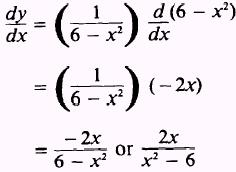Custom SearchNATURAL LOGARITHMIC FUNCTIONS Theorem 8. The natural logarithm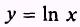has the derivative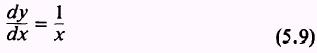for x > 0. If u is a positive differentiable function of x, then by (5.9) and the chain rule,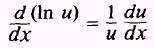EXAMPLE: Find the derivative of the function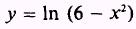SOLUTION: